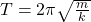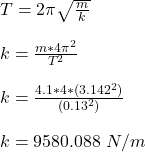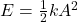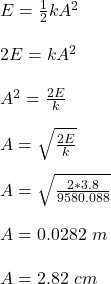## I attach a 4.1 kg block to a spring that obeys Hooke’s law and supply 3.8 J of energy to stretch the spring. I release the block and it osci

Question

I attach a 4.1 kg block to a spring that obeys Hooke’s law and supply 3.8 J of energy to stretch the spring. I release the block and it oscillates with a period of 0.13 s. What is the amplitude of oscillation

in progress 0
5 months 2021-08-17T11:56:24+00:00 1 Answers 0 views 0

The amplitude of the oscillation is 2.82 cm

Explanation:

Given;

mass of attached block, m = 4.1 kg

energy of the stretched spring, E = 3.8 J

period of oscillation, T = 0.13 s

First, determine the spring constant, k;where;

T is the period oscillation

m is mass of the spring

k is the spring constantNow, determine the amplitude of oscillation, A;where;

E is the energy of the spring

k is the spring constant

A is the amplitude of the oscillationTherefore, the amplitude of the oscillation is 2.82 cm